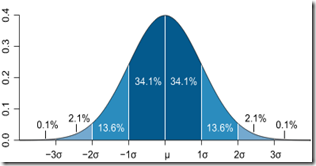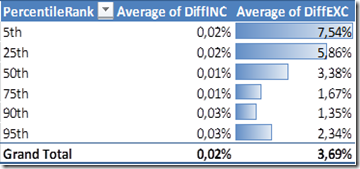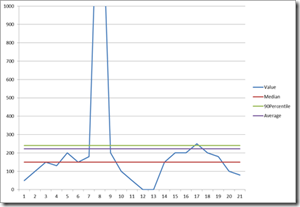## Sunday, August 29, 2010

### Percentile in PowerPivot DAXWhen analyzing data, I often find myself computing the percentile value. As I am a big fan of PowerPivot, I went with it lately to analyze some data and tried to get the 90th percentile of my series. So I chose to create a DAX measure and went looking for the PERCENTILE function only to discover that: Normal Distribution courtesy of Peter Strandmark @ Wikipedia

PowerPivot DAX doesn't support PERCENTILE! Even more shocking, neither is the much more common MEDIAN measure supported.

Don't panic folks; Marco Russo was kind enough to share his way of computing the median. With a tiny tweak it allows us to compute the 90th percentile as such:

 ```MINX(FILTER(VALUES(MyDataSerie1[Col1]), CALCULATE(COUNTROWS(MyDataSerie1), MyDataSerie1[Col1] <= EARLIER(MyDataSerie1[Col1]) ) > COUNTROWS(MyDataSerie1)*0.9), MyDataSerie1[Col1])```

The important part is 0.9. Just replace it with 0.5 for the median, 0.25 for the first quartile and so forth.

I bet the Excel and SQL folks had good reasons not to include these functions, if you have any idea why, leaves me a message. I also posted a line on the MSDN forum(http://social.msdn.microsoft.com/Forums/en/sqlkjpowerpivotforexcel/thread/b1ccf0e6-d5d3-4405-9748-4381a310b57b ).

#### Accuracy of this measure

I am no statistician, the formula looked ok but I wanted more assurance about its accuracy. Thus I fixed a little test to compare the percentile computed with this DAX measure to regular Excel PERCENTILE.INC and PERCENTILE.EXC formula. I computed the 5,25,50,75,90,95 percentiles using these 3 means for 3 different kind of number series and then averaged the difference between those :

 DiffINC is the difference in percentage between PERCENTILE.INC and the DAX measure. Same for DiffEXC.As you can see, this DAX measure is giving results pretty close (less than 0,05% error) to the PERCENTILE.INC Excel formula. This is close enough for my needs anyway. Yet be careful, with smaller number series (less than 50 items), the error went up to reach values as high as 5% on some cases.

#### Percentile Explanation

By the way, for those unfamiliar with percentile, the Nth percentile is the value below which N% of the value fall. More details on Wikipedia.Ok, so why it is useful you might ask, well it is common to analyze your data series without the extreme values that might indicate problem with your data source. An example is in the software performance testing field where you will usually consider the 90th or 95th values of the response time in order to know what the reasonable highest response time is without considering test aberrations or errors.

N.B: Some of you might have noticed the new addition to the title of this blog: Jonathan's IT blog – SharingPointers, tell me what you think in the comments !

PAC said...

I would have voted for SharingMathers seeing this post.
;-)

Jelle-Jeroen Lamkamp said...

At my office they were complaining that this was not a interpolated solution and they wanted the same results as the PERCENTILE function in excel.

So I created 3 measures iso one

Measure 1:

=MINX(FILTER(VALUES(SampleData[Interger_Value]),
CALCULATE(COUNTROWS(SampleData),
SampleData[Interger_Value] <= EARLIER(SampleData[Interger_Value]) )
> COUNTROWS(SampleData)*[Measure 8]),
SampleData[Interger_Value])

Measure 3:

=MINX(FILTER(VALUES(SampleData[Interger_Value]),CALCULATE(COUNTROWS(SampleData),SampleData[Interger_Value] <= EARLIER(SampleData[Interger_Value]) )>(COUNTROWS(SampleData)*[Measure 8])-1),SampleData[Interger_Value])

Measure 4:

=MOD(COUNTROWS(SampleData)*[Measure 8],1)+(1-[Measure 8])

If you now use Measure 4 iso your original one you got the interpolated solutionron omegna said...

hi jonathan,
I found you post on generating a 'median' calc in dax.
ok, I am truly a newbie on powerpivot and dax. so please be patient.
I have 7000 rows of data, with 2 columns, a name and a number ranging from 1-100. there are NO name duplicates. in the data model:
- I added a 3-tier row hierarchy, and
- I inserted a function, and typed your formula as posted. I hit enter, it calc'd a bit, and returned all blanks.

also, in the excel portion of the workbook, I added a pivot table. I pivot the 3-tier row hierarchy and it accurately displays levels of 'averages' of the number field. I was hoping to replace the tiered averages with tiered medians [assume that the 'median' of a data row in the lowest tier is it's same value?]

but, blanks.
ok, so I added a calculated column to find a max of the data values, no problem, pastes in the max 7000 times.
what am I missing with minx vs simple max?
I clearly am doing something simple but wrong.

thanks,
ron

Joe said...

Hello Ron,

Surprisingly I had the same results that you described when taking my original formula as such. I worked a bit on it and came up with a few changes, to sum it up we need to make sure to remove the context filters with a couple of ALL. So here is an updated version

=MINX(
FILTER(
ALL(Tableau2),
CALCULATE(COUNTROWS(Tableau2), FILTER(ALL(Tableau2),Tableau2[Value] <= EARLIER(Tableau2[Value])) ) >= COUNTROWS(Tableau2)*0.9)
, Tableau2[Value])

Tell me if it doesn't work for you, maybe with a sample of your data.

Jonathan